##### Microeconomics For Dummies - UK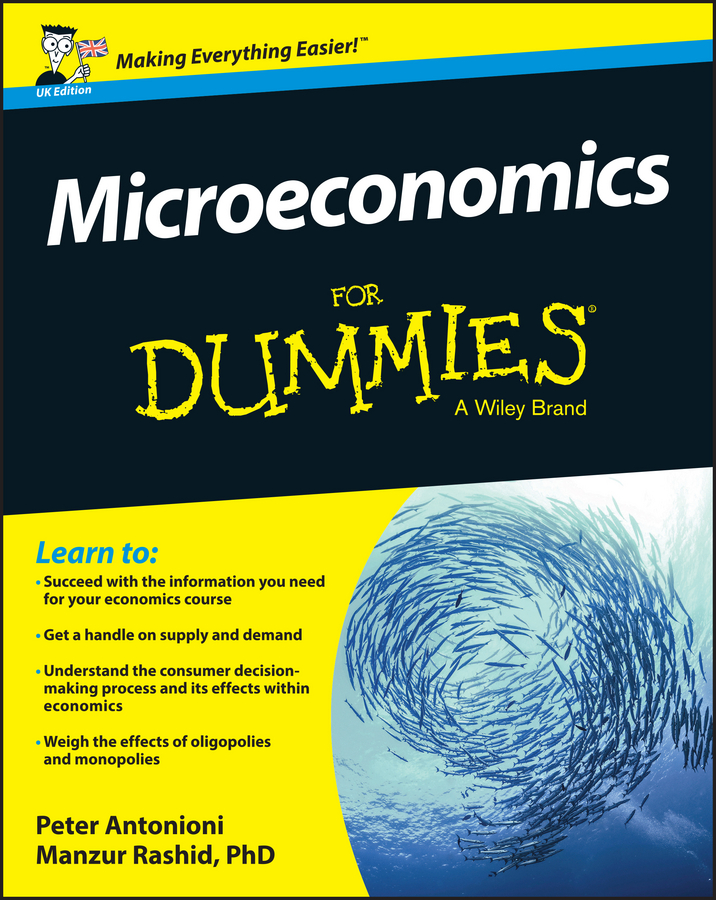Profit maximization depends on producing a given quantity of output at the lowest possible cost, and the long-run equilibrium in perfect competition requires zero economic profit. Therefore, firms ultimately produce the output level associated with minimum long-run average total cost.

Marginal cost must pass through the minimum point of average total cost. Therefore, in the long-run equilibrium, price equals three costs: minimum long-run average total cost, LRATC; the minimum point on one short-run average-total-cost curve, SRATC; and marginal cost, MC.

The illustration shows the long-run equilibrium in perfect competition. The left diagram illustrates the equilibrium price, PE, being determined by the intersection of demand and supply in the market. The perfectly competitive firm is a price taker, so this price is the firm’s marginal revenue curve, P = MR = d, in the right diagram.

This price also corresponds to minimum long-run average total cost to ensure zero economic profit in the long run. Thus, new firms have no incentive to enter the market, and existing firms have no incentive to leave the market. Price or marginal revenue equals marginal cost at q0, ensuring that profit is maximized.

Assume the short-run average-total-cost function associated with minimum long-run average total cost is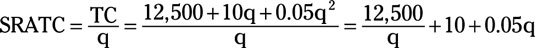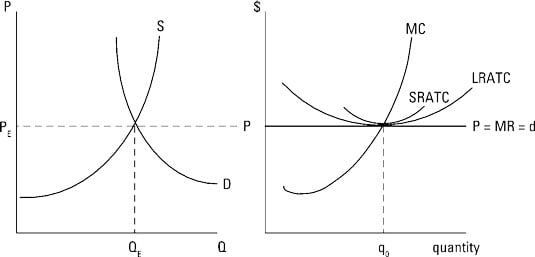The long-run equilibrium requires that both average total cost is minimized and price equals average total cost (zero economic profit is earned). In order to find the long-run quantity of output produced by your firm and the good’s price, you take the following steps:

1. Take the derivative of average total cost.

Remember that 12,500/q is rewritten as 12,500q-1 so its derivative equals –12,500q-2 or 12,500/q2.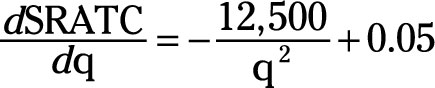2. Set the derivative equal to zero and solve for q.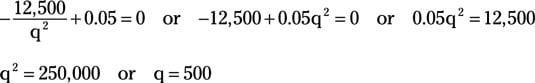or average total cost is minimized at 500 units of output.

3. Determine the long-run price.

Remember that zero economic profit means price equals average total cost, so substituting 500 for q in the average-total-cost equation equals price.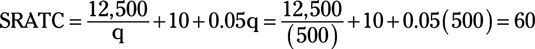The long-run equilibrium price equals \$60.00. So the firm earns zero economic profit by producing 500 units of output at a price of \$60 in the long run.

Firms have no difficulty moving into or out of a perfectly competitive market. If economic profit is greater than zero, your business is earning something greater than a normal return. This profit attracts other firms to enter the market. This situation is illustrated below.

At the initial price PA, your firm maximizes profits at qA based on marginal revenue equals marginal cost. At qA, your firm earns positive profit because price is greater than average total cost. This profit provides incentive for new firms to enter the market, increasing the market supply from SA to SLR.

The entry of new firms results in a lower equilibrium price for the good, PLR. As price decreases, your profit-maximizing quantity moves to qLR and your economic profit moves toward zero because price now equals average total cost. When economic profit reaches zero, no one has any incentive for entry or exit.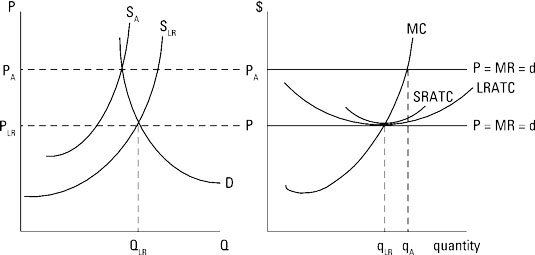Similarly, if initial economic losses exist, firms leave the market, moving the perfectly competitive market to its long-run equilibrium. The loss of firms decreases supply from SB to SLR, resulting in upward pressure on price, from PB to PLR. With the increase in price, your firm’s profit-maximizing quantity increases from qB to qLR, and zero economic profit is reached because the price PLR now equals average total cost.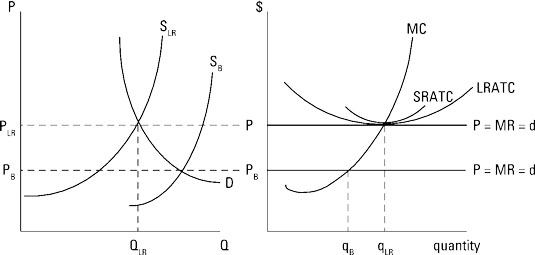So no matter where you start, in perfect competition your economic profit ultimately becomes zero. And at this point, Yogi Berra might say it’s over.